## Abstract

The `corrgram` package is an implementation of correlograms. This vignette reproduces most of the figures in Friendly (2002).

## Setup

``````library("knitr")
opts_chunk\$set(fig.align="center", fig.width=6, fig.height=6)
options(width=90)``````

The data are 11 measures of performance and salary for 263 baseball players in the 1986 baseball season in the United States. The data were used in 1988 Data Expo at the Joint Statistical Meetings.

The first 6 rows of the data and the upper-left corner of the correlation matrix are given below.

``````library("corrgram")
``````##             Name League Team Position Atbat Hits Homer Runs RBI Walks Years Atbatc Hitsc
## 1 Andy Allanson       A  CLE       C    293   66     1   30  29    14     1    293    66
## 2 Alan Ashby          N  HOU       C    315   81     7   24  38    39    14   3449   835
## 3 Alvin Davis         A  SEA       1B   479  130    18   66  72    76     3   1624   457
## 4 Andre Dawson        N  MON       OF   496  141    20   65  78    37    11   5628  1575
## 5 A Galarraga         N  MON       1B   321   87    10   39  42    30     2    396   101
## 6 A Griffin           A  OAK       SS   594  169     4   74  51    35    11   4408  1133
##   Homerc Runsc RBIc Walksc Putouts Assists Errors Salary   logSal
## 1      1    30   29     14     446      33     20     NA       NA
## 2     69   321  414    375     632      43     10    475 2.676694
## 3     63   224  266    263     880      82     14    480 2.681241
## 4    225   828  838    354     200      11      3    500 2.698970
## 5     12    48   46     33     805      40      4     92 1.963788
## 6     19   501  336    194     282     421     25    750 2.875061``````
``round(cor(baseball[, 5:14], use="pair"),2)``
``````##        Atbat Hits Homer Runs  RBI Walks Years Atbatc Hitsc Homerc
## Atbat   1.00 0.97  0.59 0.91 0.82  0.67  0.05   0.24  0.25   0.24
## Hits    0.97 1.00  0.56 0.92 0.81  0.64  0.04   0.23  0.26   0.20
## Homer   0.59 0.56  1.00 0.65 0.86  0.48  0.12   0.22  0.22   0.49
## Runs    0.91 0.92  0.65 1.00 0.80  0.73  0.00   0.19  0.20   0.23
## RBI     0.82 0.81  0.86 0.80 1.00  0.62  0.15   0.29  0.31   0.44
## Walks   0.67 0.64  0.48 0.73 0.62  1.00  0.14   0.28  0.28   0.33
## Years   0.05 0.04  0.12 0.00 0.15  0.14  1.00   0.92  0.90   0.73
## Atbatc  0.24 0.23  0.22 0.19 0.29  0.28  0.92   1.00  1.00   0.80
## Hitsc   0.25 0.26  0.22 0.20 0.31  0.28  0.90   1.00  1.00   0.78
## Homerc  0.24 0.20  0.49 0.23 0.44  0.33  0.73   0.80  0.78   1.00``````

## Figure 2

Figure 2 shows two ways to graphically display the correlation matrix using the `panel.shade()` and `panel.pie()` functions.

``````vars2 <- c("Assists","Atbat","Errors","Hits","Homer","logSal",
"Putouts","RBI","Runs","Walks","Years")
corrgram(baseball[,vars2], order=TRUE,
main="Baseball data PC2/PC1 order",
diag.panel=panel.minmax, text.panel=panel.txt)``````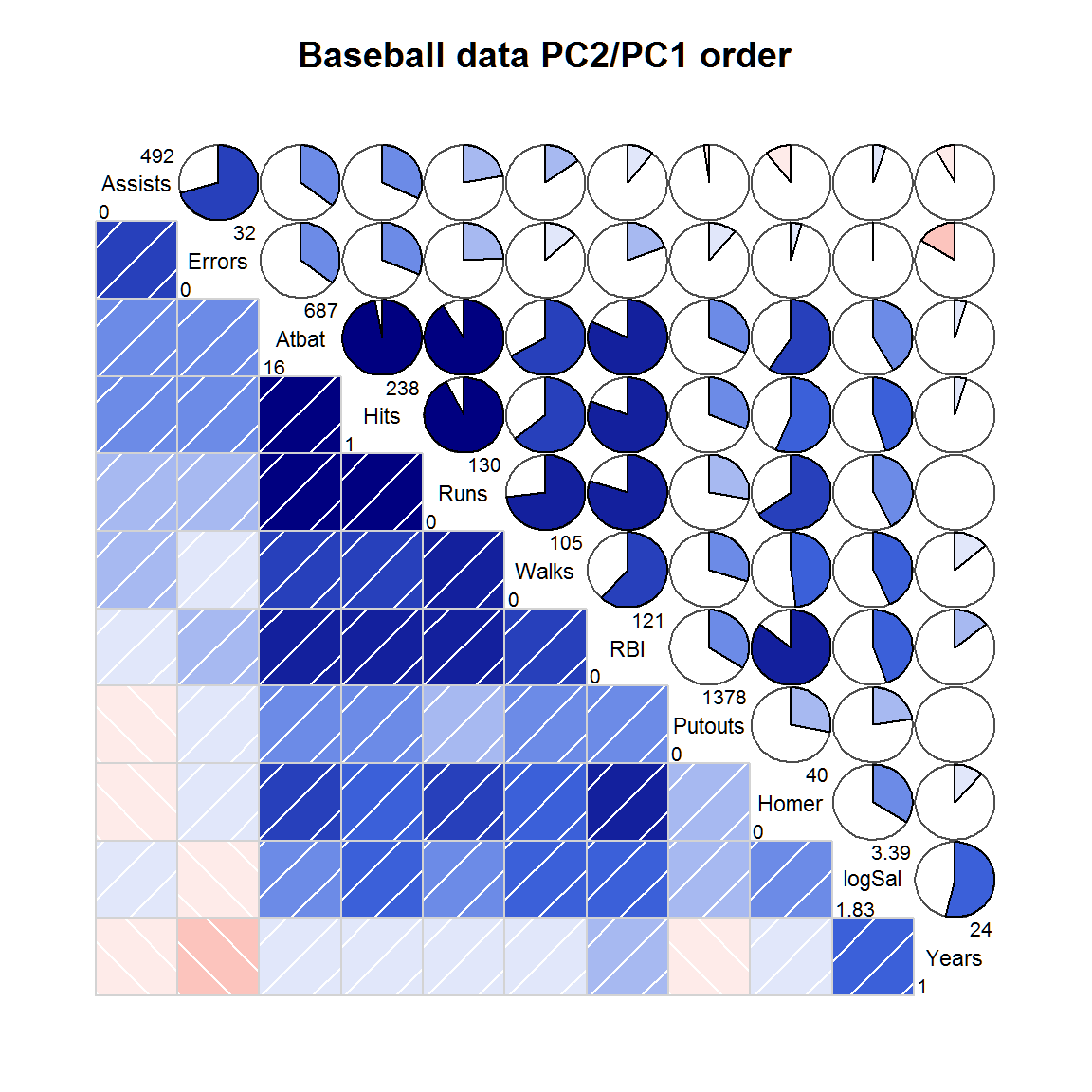## Figure 3

Figure 3 shows an eigenvector plot of the correlation matrix. This forms the basis of the orderings of the variables in the corrgram in the next section.

``````baseball.cor <- cor(baseball[,vars2], use='pair')
baseball.eig <- eigen(baseball.cor)\$vectors[,1:2]
e1 <- baseball.eig[,1]
e2 <- baseball.eig[,2]
plot(e1,e2,col='white', xlim=range(e1,e2), ylim=range(e1,e2))
text(e1,e2, rownames(baseball.cor), cex=1)
title("Eigenvector plot of baseball data")
arrows(0, 0, e1, e2, cex=0.5, col="red", length=0.1)``````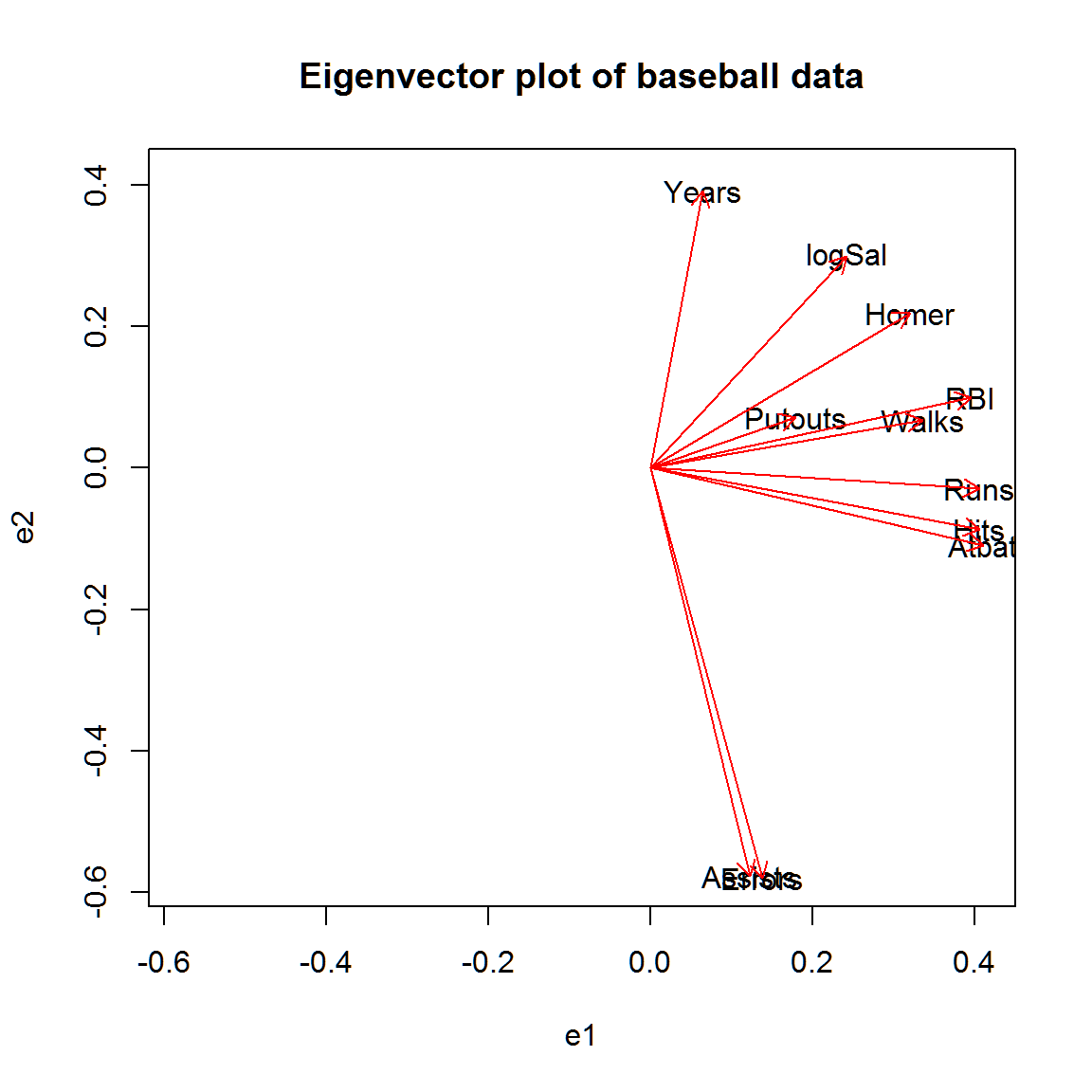## Figure 4a, 4b

In figure 4a the variables are sorted in the order as given in the data. In figure 4b, the variables are sorted according to a principal component ordering to look for possible clustering of the variables. It is not surprising to see that more times at bat is strongly correlated with a higher number of hits and a higher number of runs.

``corrgram(baseball[,vars2], main="Baseball data (alphabetic order)")``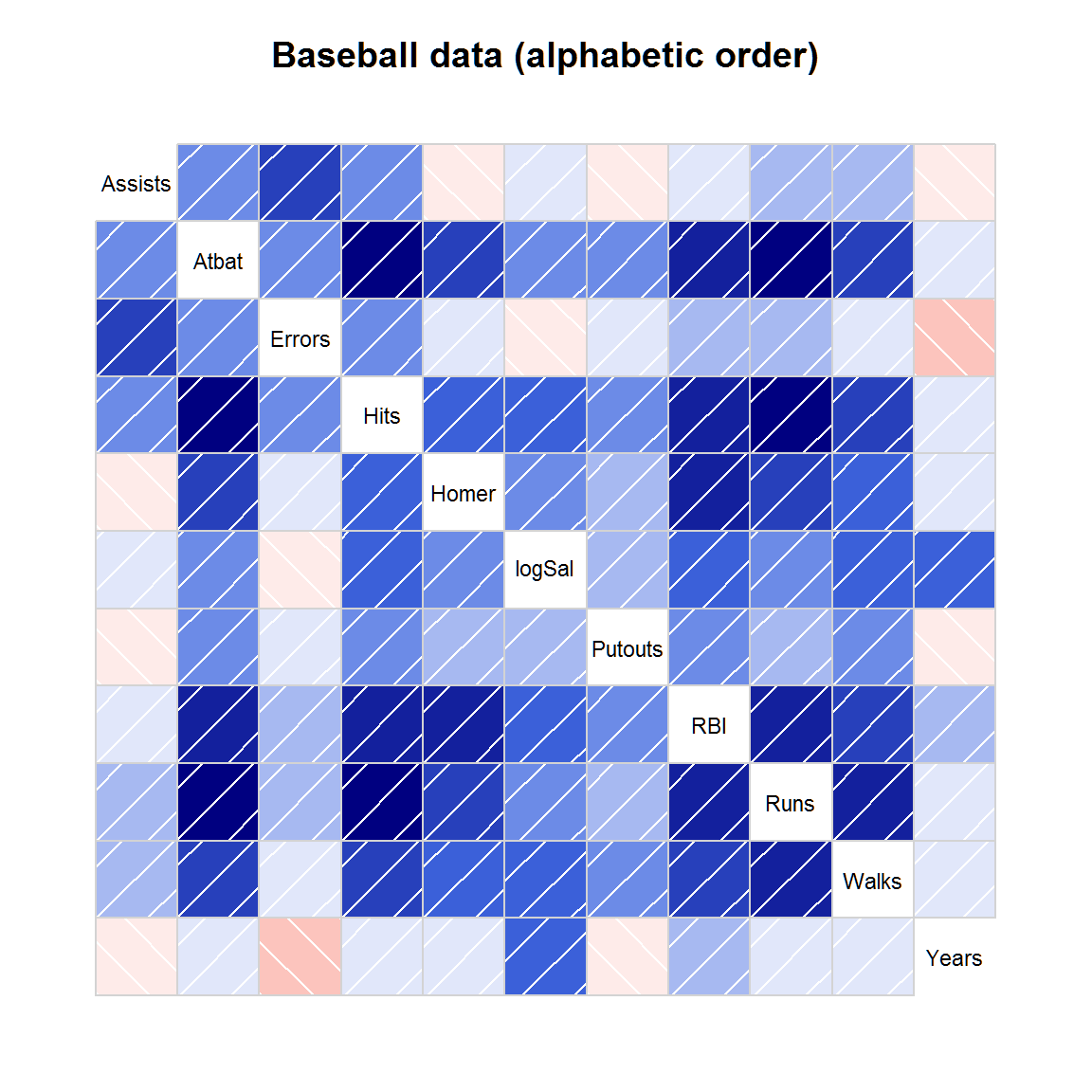``````corrgram(baseball[,vars2], order=TRUE,
main="Baseball data (PC order)",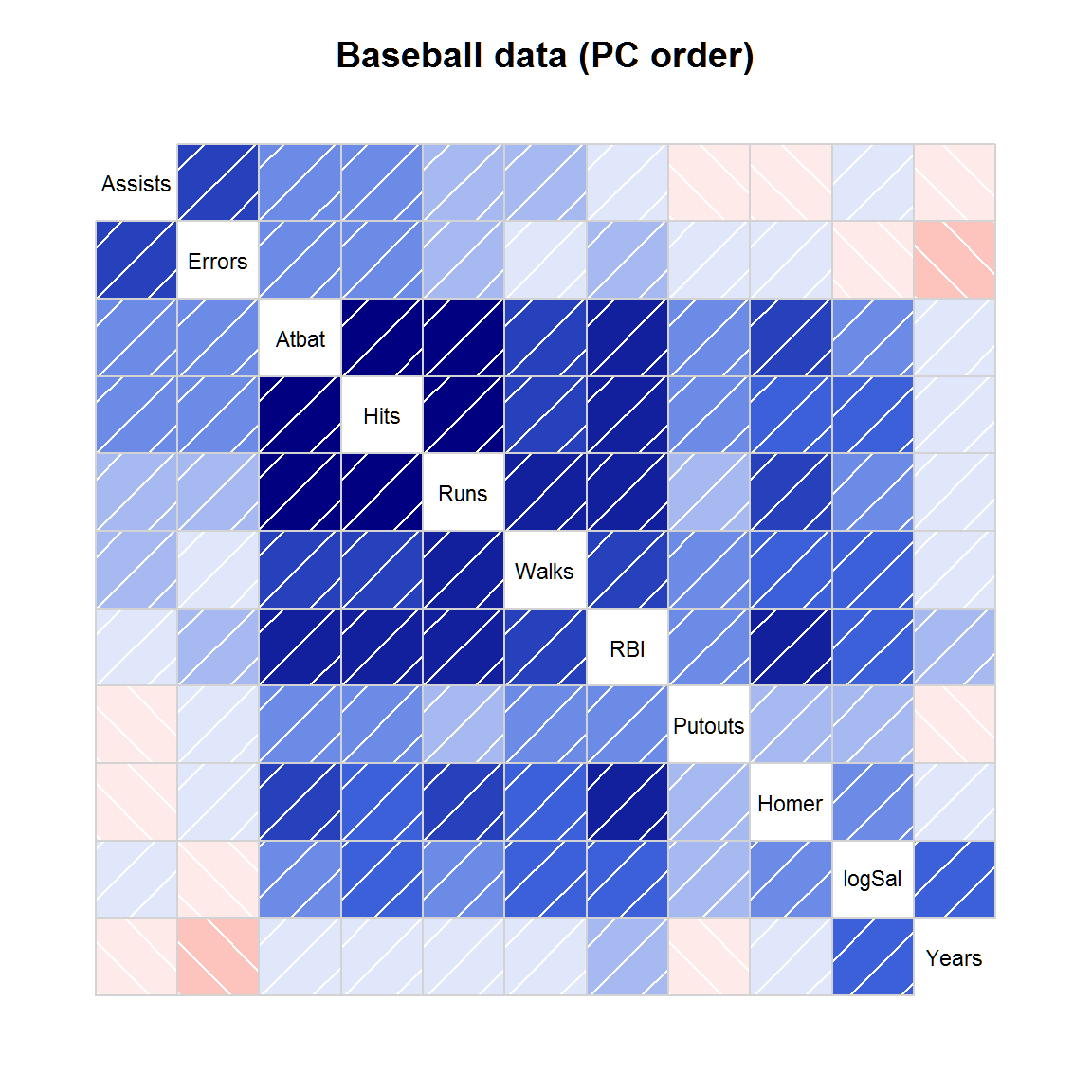## Figure 5

Figure 5 shows a corrgram for all numeric variables in the dataframe. Non-numeric columns in the data are ignored.

``corrgram(baseball, order=TRUE, main="Baseball data (PC order)")``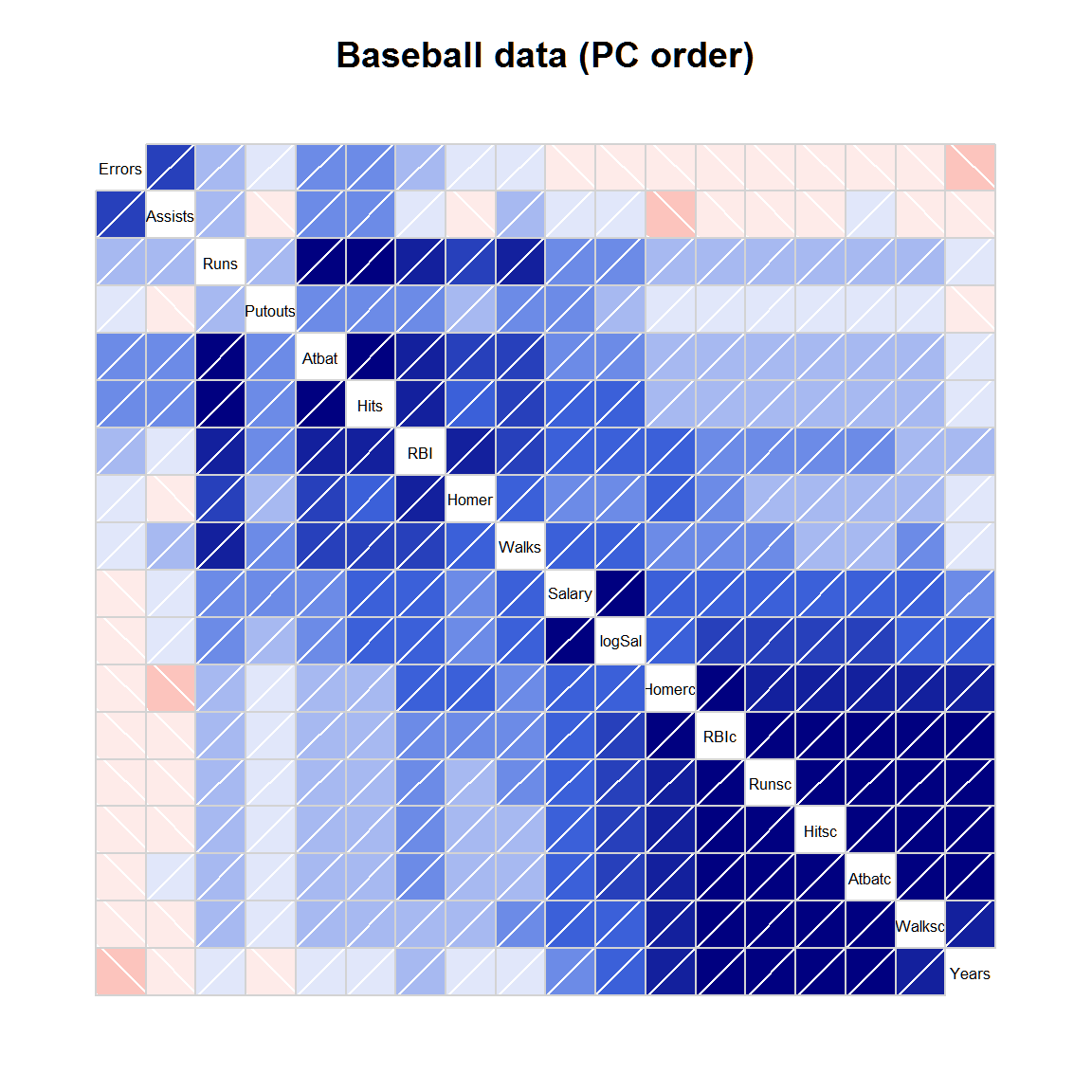## Figure 6.

Figure 6 shows a corrgram of automotive data on 74 different models of cars from 1979. There are two obvious groups of variables

Note, the arrangement is slightly different from Friendly.

``corrgram(auto, order=TRUE, main="Auto data (PC order)")``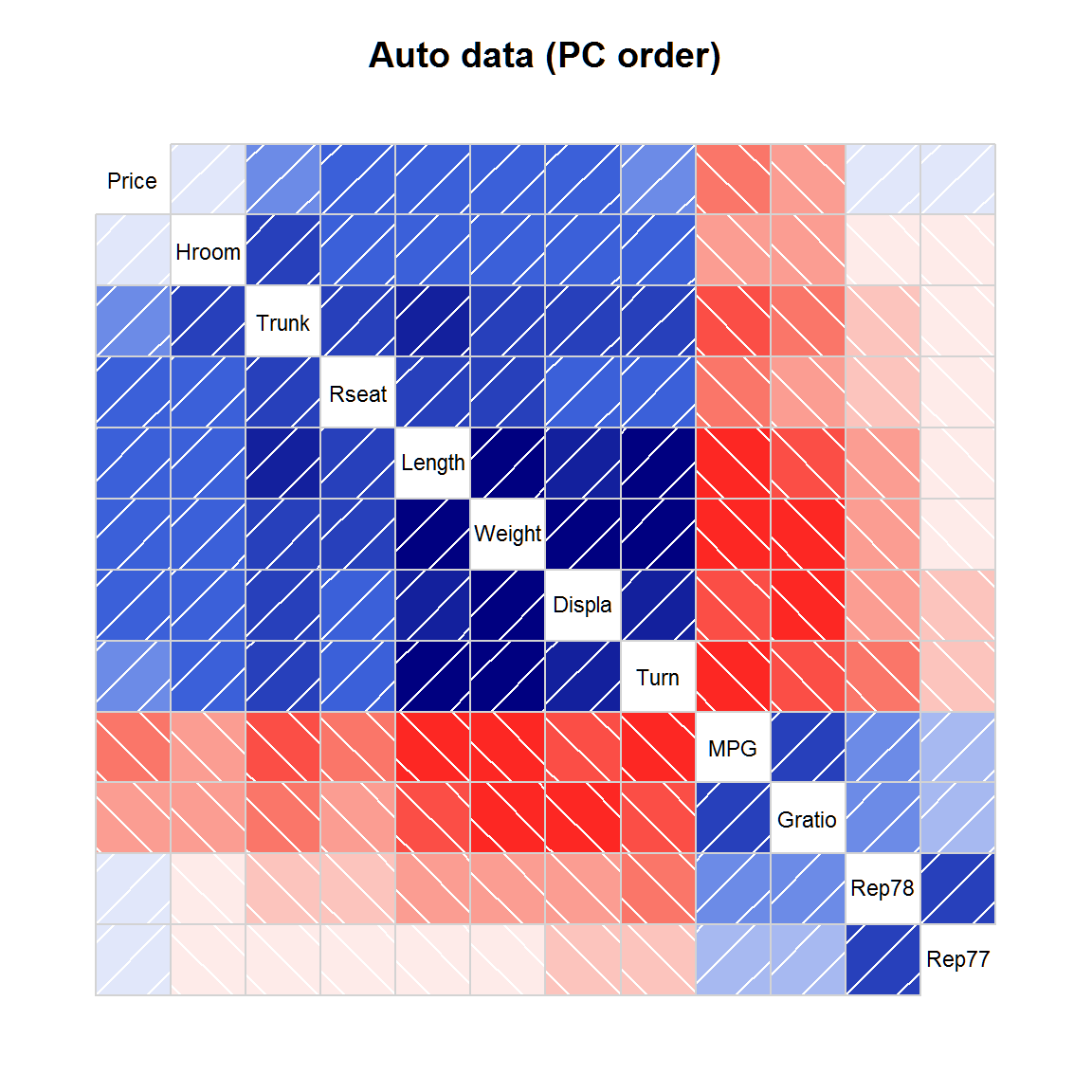## Figure 7.

The inverse of the correlation matrix expresses conditional dependence and independence of the variables.

The variables are sorted in the same order as in figure 4. One example interpretation is: controlling for all other variables, there is still a large correlation between Years and log Salary.

``````rinv <- function(r){
# r is a correlation matrix
# calculate r inverse and scale to correlation matrix
# Derived from Michael Friendly's SAS code

ri <- solve(r)
s <- diag(ri)
s <- diag(sqrt(1/s))
ri <- s %*% ri %*% s
n <- nrow(ri)
ri <- ri * (2*rep(1,n) - matrix(1, n, n))
diag(ri) <- 1  # Should already be 1, but could be 1 + epsilon
colnames(ri) <- rownames(ri) <- rownames(r)
return(ri)
}

vars7 <- c("Years", "logSal", "Homer", "Putouts", "RBI", "Walks",
"Runs", "Hits", "Atbat", "Errors", "Assists")
cb <- cor(baseball[,vars7], use="pair")
corrgram(-rinv(cb), main=expression(paste("Baseball data ", R^-1)))``````